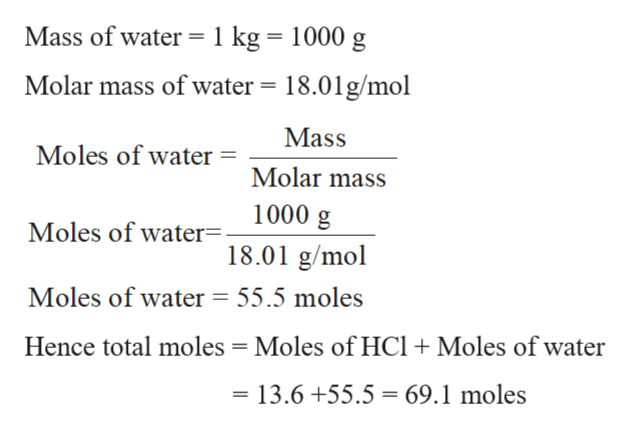# The molality of hydrochloric acid, HCl, in an aqueous solution is 13.6mol/kg. What is the mole fraction of hydrochloric acid in the solution? Answer: ______

Question

The molality of hydrochloric acidHCl, in an aqueous solution is 13.6mol/kg.

What is the mole fraction of hydrochloric acid in the solution? Answer: ______

check_circleExpert Solution
Step 1

Molality is the way to express the concentration of any solution. It can be defined as the number of moles of solute dissolves in 1 kg of the solvent. The mathematical expression for molality can be written as:

Step 2

13.6 m solution or 13.6 mol/kg aqueous solution of HCl means 13.6 moles of HCl dissolve in 1 kg of water.

Hence moles of HCl = 13.6 moles

Calculate moles of water and total moles in solution:help_outlineImage TranscriptioncloseMass of water 1 kg = 1000 g Molar mass of water 18.01g/mol Mass Moles of water Molar mass 1000 g Moles of water. 18.01 g/mol Moles of water = 55.5 moles Hence total moles Moles of HCl +Moles of water 13.6 +55.5 69.1 moles fullscreen
Step 3

Mole fraction of a component in a solution is the ratio of moles of that component and total...

### Want to see the full answer?

See Solution

#### Want to see this answer and more?

Solutions are written by subject experts who are available 24/7. Questions are typically answered within 1 hour*

See Solution
*Response times may vary by subject and question
Tagged in

### Solutions